### Select the month to view contest pictures:

February March April May June July August September October November December January

## Hunting Photo Contest Entries - November

Stand in AWECurrent Rating: 4.83
Rating (1=low, 5=high):

10 pt Whitetail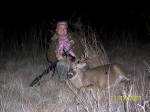Current Rating: 4.81
Rating (1=low, 5=high):

Wild Boar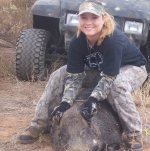Current Rating: 4.73
Rating (1=low, 5=high):Current Rating: 4.71
Rating (1=low, 5=high):Current Rating: 4.71
Rating (1=low, 5=high):

Montana duck huntingCurrent Rating: 4.71
Rating (1=low, 5=high):

Deep ThinkerCurrent Rating: 4.67
Rating (1=low, 5=high):

Group of Happy HuntersCurrent Rating: 4.64
Rating (1=low, 5=high):

My Big Buck 2010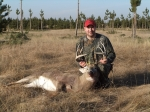Current Rating: 4.63
Rating (1=low, 5=high):

1st Whitetail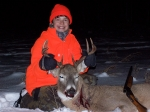Current Rating: 4.5
Rating (1=low, 5=high):

Quality TimeCurrent Rating: 4.4
Rating (1=low, 5=high):

A Moment of Peace.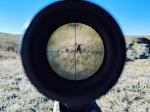Current Rating: 4.38
Rating (1=low, 5=high):Current Rating: 4.33
Rating (1=low, 5=high):

Snows in the SnowCurrent Rating: 4.17
Rating (1=low, 5=high):

Albino Triplet Does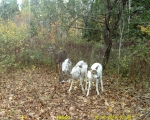Current Rating: 4.09
Rating (1=low, 5=high):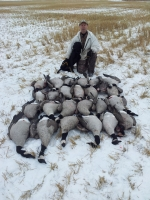Current Rating: 4
Rating (1=low, 5=high):

two fist fulls of mallardsCurrent Rating: 4
Rating (1=low, 5=high):

Late Season Mallards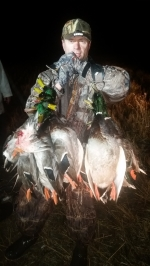Current Rating: 4
Rating (1=low, 5=high):

Pheasant Opening Day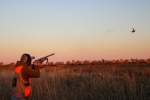Current Rating: 4
Rating (1=low, 5=high):Current Rating: 3.8
Rating (1=low, 5=high):

Yikes!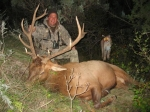Current Rating: 3.5
Rating (1=low, 5=high):

Quinn with some greenwing teal loveCurrent Rating: 3.5
Rating (1=low, 5=high):

The Endless Wait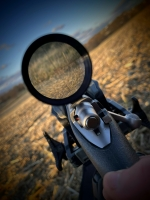Current Rating: 3
Rating (1=low, 5=high):

Duck LimitCurrent Rating: 3
Rating (1=low, 5=high):

Opening DayCurrent Rating: 3
Rating (1=low, 5=high):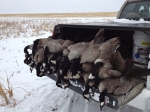Current Rating: 3
Rating (1=low, 5=high):

Where the does are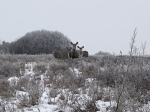Current Rating: 3
Rating (1=low, 5=high):

CallingCurrent Rating: 3
Rating (1=low, 5=high):

In search of does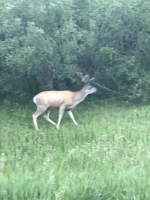Current Rating: 3
Rating (1=low, 5=high):

staking the decoy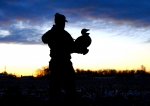Current Rating: 3
Rating (1=low, 5=high):

A Moment of Peace.Current Rating: 3
Rating (1=low, 5=high):

Another day, another limitCurrent Rating: 3
Rating (1=low, 5=high):

4x4 muley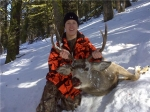Current Rating: 2.8
Rating (1=low, 5=high):

WidgeonCurrent Rating: 2.5
Rating (1=low, 5=high):